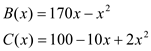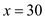## Quiz 3 : Marginal Analysis for Optimal DecisionsLooking for Economics Homework Help?# Quiz 3 : Marginal Analysis for Optimal Decisions

Optimization theory finds the optimal or best solution by weighing the objective with its cost and benefits. a. The optimal number of traffic accidents is zero represents minimization problem. The objective is to minimize the traffic accidents. However, zero traffic accidents might represent low or no car movement on roads which is imaginary. b. Any pollution is too much pollution refers to minimization problem. The aim is minimize or have nil pollution. c. The decision not to send more troops despite requirement due to constraint of sending troops to Country A refers the expenses that cannot be recovered once put. These types of costs are sunk cost. d. Continuing to spend due to non-recovery of previous costs invested refers again to sunk cost. The decision to continue depends on the previous cost. d. The net benefit would increase as a result of increase in small cost. This is because the benefits would be greater than costs.

The equation of benefit function ( B ) and cost function ( C ) are given as,(a) Marginal benefit is the differentiated equation of total benefit equation. The following shows the marginal benefit:The marginal cost is the differentiated mount of total cost:(b) The net benefit (NB) is the difference between the total benefit (B) and total cost (C) :The level of x that maximizes net benefit function can be calculated by differentiating the net function and equating it to zero:Thus, the value of choice variable that maximizes the net benefit is 30 (c) The maximum level of net benefit can be calculated by substituting the value ofin net benefit ( NB ) equation:Thus, the maximum level of net benefit is 2,600

The optimization problem aims at maximizing the net benefit. The optimal point can be calculated by equating the marginal cost (MC = 40P) with marginal benefit or revenue (MR = 1,000 - 10P).Therefore, 20 units of pollution should be reduced in order to minimize the cost and maximize the revenue.